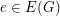# Bouchet's 6-flow conjecture

 Importance: High ✭✭✭
 Author(s): Bouchet, Andre
 Subject: Graph Theory » Coloring » » Nowhere-zero flows
 Keywords: bidirected graph nowhere-zero flow
 Prize: none
 Posted by: mdevos on: March 7th, 2007
Conjecture   Every bidirected graph with a nowhere-zero-flow for some, has a nowhere-zero-flow.

Definition: A bidirected graph is a graph in which every edge has two arrowheads, one next to each endpoint. If the edgehas endsand, then the arrowheads nearestandmay point either towardor toward(giving four possibilities in all). Ifis a bidirected graph, a-flow of G is a mapwith the property that at every vertex, the sum ofon the edges whose ends atare directed intois equal to the sum ofon the edges whose ends atare directed out of. We say thatis nowhere-zero iffor every(see nowhere-zero flows).Flows on bidirected graphs arise naturally as duals of local-tensions on a non-orientable surface. For more on this relationship, see [B]. Bouchet proved that the above conjecture is true with 6 replaced by 216, and exhibited a bidirected Petersen graph as above which shows that 6 is the best value possible. Zyka [Z] and independently Fouquet improved upon this result proving that the above conjecture is true with 6 replaced by 30. Khelladi [K] proved that for 4-connected graphs, the above conjecture is true with 6 replaced by 18. DeVos [D] proved that the above conjecture holds with 6 replaced by 12, and showed that every 4-edge-connected bidirected graph with a nowhere-zero integer flow also has a nowhere-zero 4-flow.

## Bibliography

[B] A. Bouchet, Nowhere-Zero Integral Flows on a Bidirected Graph, J. Combinatorial Theory Ser. B 34 (1983) 279-292. MathSciNet

[D] M. DeVos, Flows on Bidirected Graphs, preprint.

[K] A. Khelladi, Nowhere-Zero Integral Chains and Flows in Bidirected Graphs, J. Combinatorial Theory Ser. B 43 (1987) 95-115. MathSciNet

[Z] O. Zyka, Bidirected Nowhere-Zero Flows, Thesis, Charles University, Praha (1988).

* indicates original appearance(s) of problem.